´

The ladder game is an algorithm for the stepwise determination of transversals of the sets of double cosets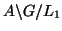to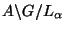for a subgroup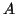of a group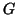and a sequence of subgroups, a so-called ladder,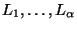ofwith either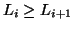or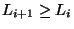for all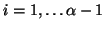.

We call a step from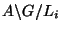to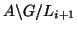witha step down. A double coset ofand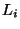splits into double cosets ofand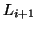: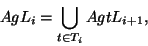for a transversal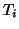of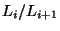. Therefore, a transversal of the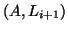-double cosets is a subset of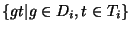where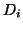means a transversal of the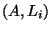-double cosets. This subset has to be determined.

A step up fromtois a step with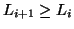. Such double cosets ofandfuse to a double coset ofand: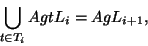for a transversalof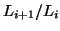. We obtain a transversal of the double cosets ofandas a subset of a transversal of the double cosets ofand.

To keep down the complexity of this algorithm, we should try to choose a ladderwith a small index betweenandfor all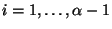, because during each step, dow or up, respectively, we run through a transversal of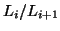and, respectively.

In order to visualize the ladder game we introduce a graph which we call orbit graph:

• The-double cosets,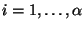, correspond to the vertices of the graph.
• Two vertices are connected by an edge iff the corresponding double cosets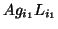and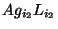fulfill
1.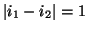2.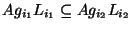or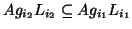.
• Each vertex is labeled by a tuple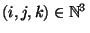, where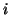means the step in the ladder game,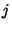is the-th double coset ofandand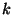is the order of the stabilizer of the corresponding double coset in.

Let us return to the determination of transversals of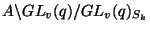and of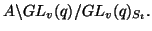We need a ladder of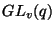containing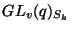and. For this purpose we take the following sequence of parabolic subgroups (generalizing this way the ladder game that we applied to designs on sets, where we took a ladder of Young subgroups!)

$$$$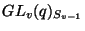$$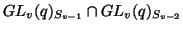$$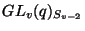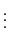$$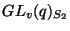$$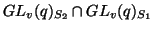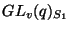as a ladder. Starting from the trivial transversal of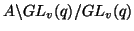we can determine step by step all transversals of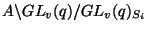for all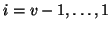.

Let us display a concrete example: We take the parameters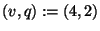and, for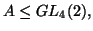we choose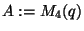, the complete monomial group, which is isomorph to the wreath product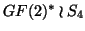. Then we get the following orbit graph: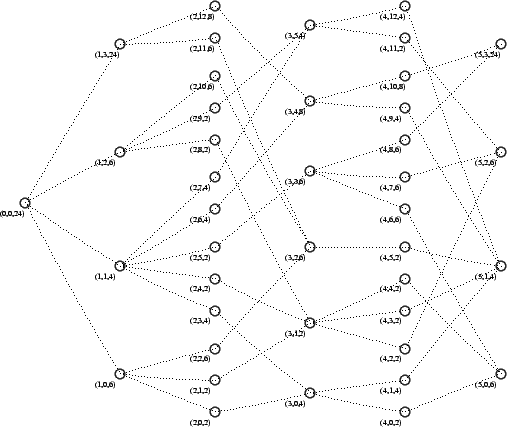In this graph the vertices with the labels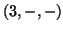correspond to the orbits of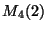on the set of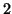-supspaces of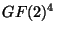and the vertices labeled by the tuples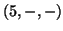correspond to the orbits ofon the set of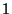-subspaces.

University of Bayreuth -